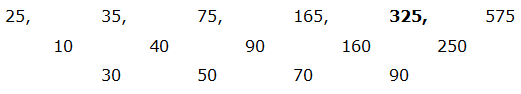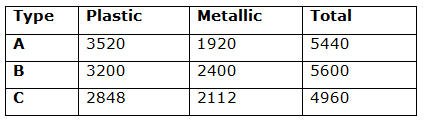# SBI Clerk Pre Quantitative Aptitude (Day-48)

Dear Aspirants, Our IBPS Guide team is providing new series of Quantitative Aptitude Questions for SBI Clerk Prelims 2020 so the aspirants can practice it on a daily basis. These questions are framed by our skilled experts after understanding your needs thoroughly. Aspirants can practice these new series questions daily to familiarize with the exact exam pattern and make your preparation effective.

Start Quiz

Ensure Your Ability Before the Exam – Take SBI Clerk 2020 Prelims Free Mock Test

Number series

Directions (1 – 5): What value should come in the place of question mark (?) in the following number series?

1) 59, ?, 94, 158, 283

a) 52

b) 56

c) 61

d) 62

e) 67

2) 39, 26, 47, 18, ? , 10

a) 50

b) 52

c) 48

d) 55

e) 37

3) 96, 480, 120, 600, ? , 750

a) 140

b) 150

c) 100

d) 200

e) 250

4) 85, 106, 74, 119, ? , 136

a) 59

b) 65

c) 42

d) 79

e) 58

5) 25, 35, 75, 165, ?, 575

a) 200

b) 300

c) 250

d) 325

e) 425

Caselet

Directions (6 – 10): Study the following information carefully and answer the given questions?

A company manufactures three types of product viz. Product A, B, C. In the year 2018, the company manufactured a total 16000 products. Out of the total products some are of plastic material and some are of metallic material.

35% of the total products are product B. Ratio of plastic and metallic products in product B is 4: 3. Number of Plastic materials of product A is 10% more than the number of plastic products of product B. Ratio of metallic materials of product C and the number of plastic materials of product A is 3: 5 respectively. Number of Metallic materials of product A is 20% less than the number of metallic materials of product B.

6) Find the difference between the plastic materials and the metallic materials of product C?

a) 694

b) 784

c) 796

d) 736

e) 682

7) Find the respective ratio of number of metallic materials of product A and number of metallic materials of product B?

a) 1: 2

b) 3: 2

c) 4: 5

d) 2: 5

e) 5: 4

8) Find the total number of product A and product B?

a) 11000

b) 11020

c) 11080

d) 11010

e) 11040

9) Number of plastic materials of product A is approximately what percentage of total materials of product B?

a) 63 %

b) 72 %

c) 56 %

d) 44 %

e) 85 %

10) Find the difference between the Plastic materials of product B and the metallic materials of product A?

a) 1400

b) 1380

c) 1320

d) 1200

e) 1280

Directions (1-5) :

59 + 2³ = 67

67 + 3³ = 94

94 + 4³ = 158

158 + 5³ = 283

39 – 13 = 26

26 + 21 = 47

47 – 29 = 18

18 + 37 = 55

55 – 45 = 10

96 * 5 = 480

480 / 4 = 120

120 * 5 = 600

600 / 4 = 150

150 * 5 = 750

85 + 7 * 3 = 106

106 – 8 * 4 = 74

74 + 9 * 5 = 119

119 – 10 * 6 = 59

59 + 11 * 7 = 136

(Or)

The alternative difference,

85 – 11 = 74, 74 – 15 = 59

106 + 13 = 119, 119 + 17 = 136

25 + 10 * 1² = 35

35 + 10 * 2² = 75

75 + 10 * 3² = 165

165 + 10 * 4² = 325

325 + 10 * 5² = 575

(Or)The difference of difference is, 30, 50, 70, 90,…

Directions (6 – 10):

Total = 16000

35% of the total products are product B

Total products of B type = 35% 16000 = 5600

Ratio of plastic and metallic products in product B is 4:3

Plastic products of product B = 4/7 * 5600 = 3200

Metallic products of product B = 3/7 * 5600 = 2400

Plastic products of product A = 110% (number of plastic products of product B)

= > 110 % 3200 = 3520

Ratio of metallic products of product C and the number of plastic product of product A is 3:5 respectively

Metallic products of product C = 3/5 * 3520 = 2112

Number of Metallic products of product A = 80% of 2400 =1920

Total products of B = 5600

Total products of A = 3520 + 1920 = 5440

Total Products of C = 16000 – (5600 + 5440) = 4960

Total Plastic products of Product C = 4960 – 2112 = 2848Difference between the plastic materials and the metallic materials of product C

= 2848 – 2112

=736

Required Ratio = 1920: 2400 = 4: 5

Total = 5440 + 5600 = 11040

Number of plastic materials of product A = 3520

Total product of B = 5600

Required percentage = (3520 / 5600) * 100 = 62.85 % ≈ 63%